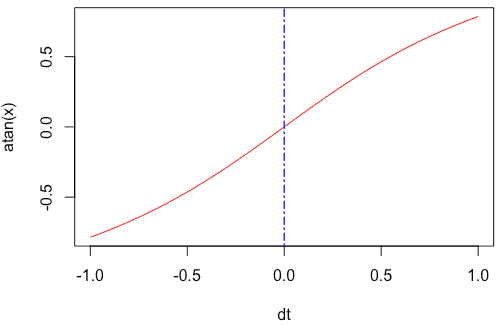# What is the atan() Function in R

The atan() function in R is “used to return inverse tangent value of a numeric value”. For example, atan(1) returns 0.7853982 radians.

### Syntax

``atan(number)``

### Parameters

number: It’s a number as a numeric value.

### Return value

The atan() function returns the inverse tangent of a number (radians) sent as a parameter. It is between [-pi/2,pi/2] in radians.

## Example 1: How to use the atan() function

``````v1 <- -1
v2 <- 0.5
v3 <- 0

atan(v1)
atan(v2)
atan(v3)``````

Output

`````` -0.7853982
 0.4636476
 0``````

If you pass the 0 to the atan() function, it will return 0.

## Example 2: Using atan() function with a Vector

To apply atan() function to a vector, you can use the c() function to create a vector and then pass it to the atan() function.

``````rv <- c(-1, 0.5, 0, 0.5, 1)
atan(rv)``````

Output

`` -0.7853982 0.4636476 0.0000000 0.4636476 0.7853982``

## Example 3: Passing a pi to the atan() function

The pi is a built-in constant in R programming, and its value is 3.141593.

Let’s find the pi constant’s atan() value.

``atan(pi)``

Output

`````` 1.262627
``````

Let’s see another example of pi.

``atan(pi / 4)``

Output

`````` 0.6657738
``````

## Plot the atan() function to a graph

We can use the seq() function to create a series of values and pass that to the plot() function, creating a line chart.

``````dt <- seq(-1, 1, by = 0.01)

plot(dt, atan(x), typ = "l", col = "red")

abline(v = 0, lty = 6, col = "blue")``````

OutputThat is it for this tutorial.